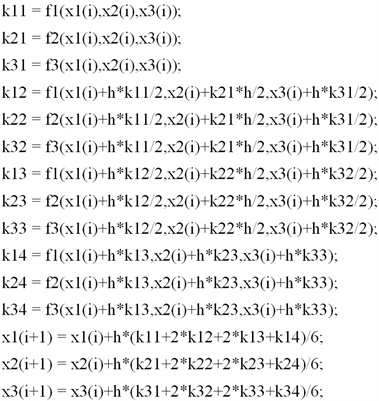# 常微分方程常用的数值算法及其应用Numerical Algorithms for Ordinary Differential Equations and Application

• 全文下载: PDF(419KB)    PP.2045-2049   DOI: 10.12677/AAM.2019.812235
• 下载量: 143  浏览量: 250   科研立项经费支持

In this paper, we mainly use MATLAB software to program the numerical solution of ordinary differential equation, such as Euler method and classical fourth-order Runge Kutta algorithm. Through the results of corresponding numerical example, we analyze the advantages and disadvantages of these two algorithms. Finally, we apply those algorithms to a concrete example; we solve it and compare the results.

1. 常用的数值算法1.1. 算法介绍1.2. 数值算例Table 1. Comparison of calculation results

2. 算法的实际应用Table 2. Calculation results of Euler methodTable 3. Calculation results of fourth-order classical Runge Kutta algorithm

3. 结论

  钟巍. 一种新的常微分方程初值问题数值解法[J]. 重庆文理学院学报(自然科学版), 2011(4): 15-18.  林爽, 张杰. 常微分方程初值问题的基本数值解法分析[J]. 海南师范大学学报(自然科学版), 2012, 25(2): 119-121.  霍晓程. 常微分方程数值解法的研究[J]. 佳木斯教育学院报, 2011(5): 167-167.  孙美玲. 常微分方程数值解法的Matlab计算与可视比较[J]. 高教学刊, 2016(19): 60-61.  余丽. 常微分方程数值解法的若干求解格式[J]. 鄂州大学学报, 2013(S1): 123-125.  孙燕新, 李娟. 关于几种常用一阶常微分方程数值解法的导出[J]. 常州工程学院报, 2010(6): 71-74.  李艳杰. 非线性时滞脉冲动力系统的辨识模型, 优化算法及应用[D]: [硕士学位论文]. 大连: 大连理工大学, 2005, 12.